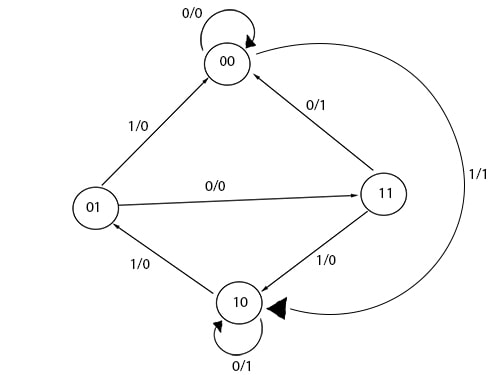### Exam Year

Tribhuvan University

Institute of Science and Technology

2078

Bachelor Level / first-semester / Science

Computer Science and Information Technology( CSC111 )

Digital Logic

Full Marks: 60 + 20 + 20

Pass Marks: 24 + 8 + 8

Time: 3 Hours

Candidates are required to give their answers in their own words as far as practicable.

The figures in the margin indicate full marks.

Section A

Attempts any two questions

1

Design the sequential circuit with respect to the following state diagram using J-K flip flops.2

Implement F = Σ(0, 2, 3, 4, 7) using

1. Multiplexer
2. Decoder
3. PLA
3

Difference between synchronous and asynchronous counter. Design mode-7 synchronous counter using T-flip flop. Show necessary truth tables and k-maps.

Section B

Attempt any two questions

4

Provide one example where shift right operation can be used. Explain parallel-in-parallel-out register.

5

1. Preform 1’s complement subtraction 110101 – 100101
2. Represent decimal number 0.125 into its binary form
6

Derive the Boolean expression for sum and carry of half adder. Draw its combinational circuit. Implement it using only NAND gates.

7

Express the Boolean function F = x + yz as product of max-terms.

8

Minimize the Boolean function Boolean function using K-map

F(A, B, C, D) = Σ(0, 1, 3, 5, 7, 8, 9, 11, 13, 15)

9

What are the practical implementations of up counter? Explain Binary ripple counter.

10

Design a combinational circuit with three inputs and one output. The output is 1 when the binary value of the inputs is an odd number.

11

Differentiate between PLA and PAL. Explain 4-bit magnitude comparator

12

Write short notes on (Any Two)

1. Negative Logic
2. CMOS
3. EBCDIC